Next: B.2 Root Finding Up: B. Ray-Isosurface Intersection Previous: B. Ray-Isosurface Intersection

# B.1 Setup of the Polynomial

Inserting the ray parameterization (B.1) into (B.4) leads to a polynomial of order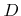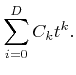(B.8)

The calculation of the coefficients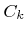described in the literature [69,74,88,119] is not optimal in terms of the number of required multiplications. Therefore, optimized algorithms, Algorithm B.2 and Algorithm B.3, have been developed for the two- and three-dimensional cases, respectively. There,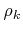denotes the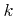-th element of the multi-linear polynomial coefficients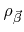, if they are sorted in lexicographical order. For example,corresponds to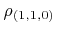in the three-dimensional case.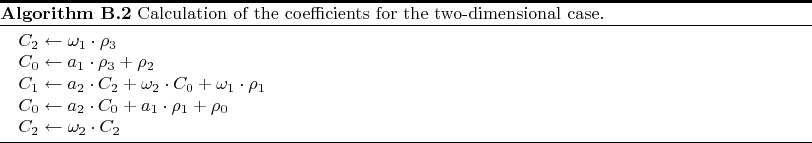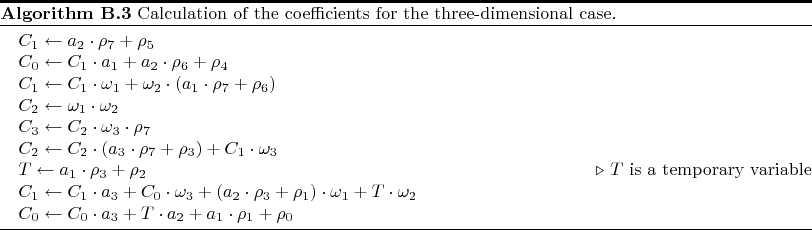Next: B.2 Root Finding Up: B. Ray-Isosurface Intersection Previous: B. Ray-Isosurface Intersection

Otmar Ertl: Numerical Methods for Topography Simulation